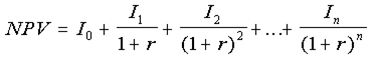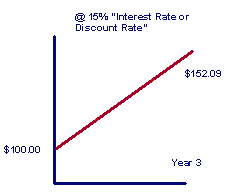# Calculating risks and measuring return on CRM software with IRR and NPV

## Read Tom Pisello's advice on calculating risks and measuring return on CRM, including advice on IRR and NPV in this tip.

Is internal rate of return (IRR) or net present value (NPV) a better metric for calculating risks and measuring return on a CRM system?
As discussed in prior Q/As, there are generally five key measures to summarize the risk and reward value of a project. They include ROI, NPV, risk-adjusted ROI, IRR and payback period. Each analyzes the cash flow of project including the costs (investments) and benefits over time. Each metric has strengths and weaknesses hence one measure is typically not enough to base a significant decision upon. In short an overall financial business case would be the best option.

The main difference between IRR and NPV is depicted in the formulas below and explained.

IRR
IRR is often used in capital budgeting. It is the interest rate that makes net present value of all cash flow equal zero. It is primarily the value another investment would need to generate in order to be equivalent to the cash flows of the investment being considered.

The discount rate (r) is necessary to drive the NPV formula to zero:The benefit to IRR is that illiterates overall returns in clear percentage terms and its great for comparing project returns head to head. However it does not indicate the level of investment required up-front or the overall dollar value of returns.

NPV
The NPV, also referred to as the time value of money, essentially measures the value today of cash received at a future date given an interest rate. The NPV of an investment tells you how an investment compares either with your alternative investment or with borrowing. A positive net present value represents an investment that may be a good option.

A negative net present value means your alternative investment, or not borrowing may be a better option.

Example:
If you have to spend \$100.00 today, you have to get \$152.09 back in three years to be equivalent at a 15% interest rate. The 15% can be viewed as a discount rate, converting the \$152.09 benefit in three years, back into \$100.00 today.Measuring NPV puts the net benefits of project into today's dollar terms. The benefits to using NPV include a true representation of savings over an analysis period. However, NPV does not highlight how long it can take to get positive cash flow and only portrays net savings not the ratio of Investments (costs) to savings.

Close